# Risk parity

Risk parity (RP) is a risk-based portfolio approach. It differs from Markowitz’s approach in that a risk parity strategy does not make use of expected returns. Instead,RP only requires the covariance matrix to construct the optimal weights. On this page, we discuss the idea behind risk parity and provide the necessary formulas to apply the approach in the case of two assets. We also illustrate how to apply the risk parity two assets case in Excel using a simple example at the bottom of this page.

## Why does risk parity work?

When applying RP, the weights of the portfolio will be determined in such a way that each asset contributes in the same way to overall portfolio volatility. Hence, instead of using an approach where we allocate the same amount of capital to each security (as is the case in an equal-weighted portfolio), we allocate the capital over the different securities so that the risk contributed by each position is the same. That is why the approach is sometimes also referred to as an equal-risk contribution portfolio approach.

## Risk parity formula

In the case of two assets, we can easily calculate the weights for the RP portfolio. In this case, we do not require a difficult numerical optimization. Given the volatility of asset 1 (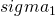) and asset 2 (), we can calculate the weights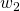and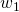as follows: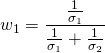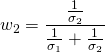## 2-asset case risk parity example

Let’s consider a simple example to illustrate how RP works. In the case of two assets, the risk parity portfolio weights can easily be determined using a set of formulas. If we have more than two assets, however, things become a bit more difficult and optimization techniques are needed.

Consider two assets, for example stocks and bonds. Using historical returns, we can estimate the risk of both securities. Suppose we calculate the standard deviation of both securities and we obtain 4.50% for asset 1 (stocks) and 1.62% for asset 2 (bonds). The weights will then be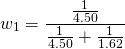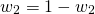Thus, 26.4% will be invested in asset 1 and 75.53% will be invested in asset 2.

## Risk parity in practice

Since a RP portfolio allocates most of the portfolio in the less risky asset class, the overall expected return of the portfolio will be lower. That’s why, in practice, the portfolio is often leveraged. Thus, the manager will borrow additional money to ensure that a certain expected return is obtained. Suppose, for example, that the expected return of the unleveraged RP portfolio is 4%. The manager however, wants to have an expected return of 7%. In that case he will leverage the overall portfolio 1.75 times (7%/4%).

## Risk parity funds

There are a number of famous hedge funds that implement trading strategies that rely on RP. One example is Bridgewater, whose All Weather portfolio, makes use of RP. Another hedge fund that employs risk parity is Applied Quantitative Research (AQR).

## Summary

Risk parity is a relatively new way of constructing portfolios that ensures that risk is evenly distributed across the securities in the portfolio. It is used by hedge funds. However, a number of risk parity ETF also exist today.

### Risk parity calculator

Want to implement a two asset risk parity portfolio in Excel? Download the Excel file: Risk Parity.